### Total 23 Videos found in Category "Trigonometry Lectures"

Title
 1Trigonometry Lectures: Addition and Subtraction Formulas 2Trigonometry Lectures: Area of a Triangle SAS 3Trigonometry Lectures: Complex Numbers (Simplify i) 4Trigonometry Lectures: Converting Between Degrees and Radians 5Trigonometry Lectures: Converting Polar Coordinates to Standard to Rectangular 6Trigonometry Lectures: DeMoivre Theorem 7Trigonometry Lectures: Double Angle Formula 8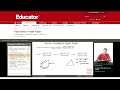Trigonometry Lectures: Find All Angles in a Right Triangle 9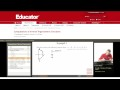Trigonometry Lectures: Find the Arcsines of Common Angles 10Trigonometry Lectures: Find the Tangent and Cotangent 11Trigonometry Lectures: Graph the Secant and Cosecant Functions 12Trigonometry Lectures: Graphing Sine and Cosine 13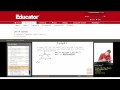Trigonometry Lectures: Law of Cosines 14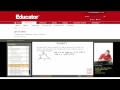Trigonometry Lectures: Law of Sines 15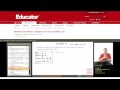Trigonometry Lectures: Modified Sine Waves 16Trigonometry Lectures: Polar Form of Complex Numbers (Multiply Two Complex Numbers) 17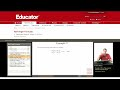Trigonometry Lectures: Prove the Half Angle Formula 18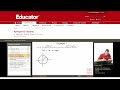Trigonometry Lectures: Prove the Pythagorean Identity 19Trigonometry Lectures: Sine and Cosine Values of Special Angles 20Trigonometry Lectures: Tangent Secant Identity (Given Tan find Sec) 21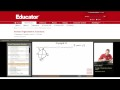Trigonometry Lectures: Values of Arcsine, Arccosine, Arctangent 22Trigonometry Lectures: Vectors (Force on a Box) 23Trigonometry Lectures: Word Problems (Bridge Across Lake)

Say and share some thing about these videos...